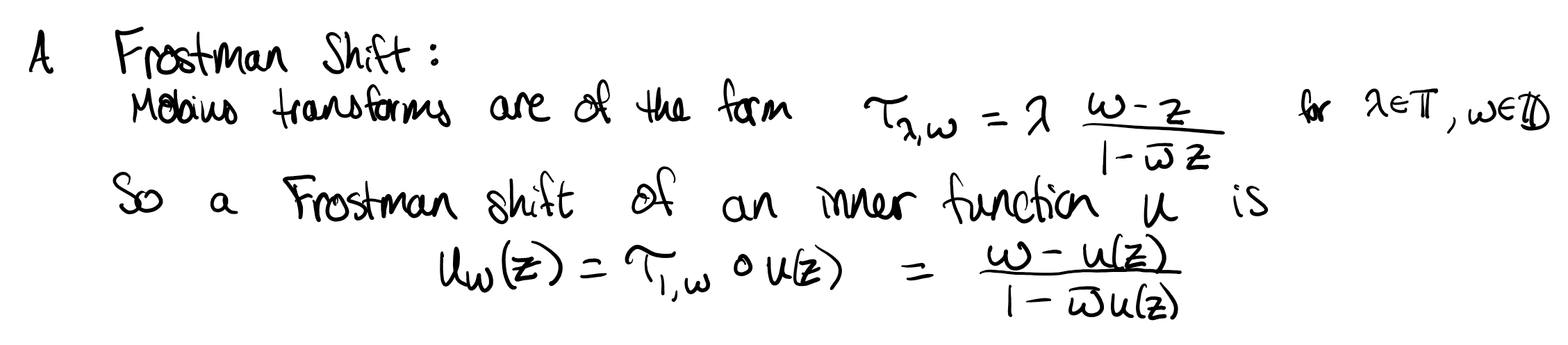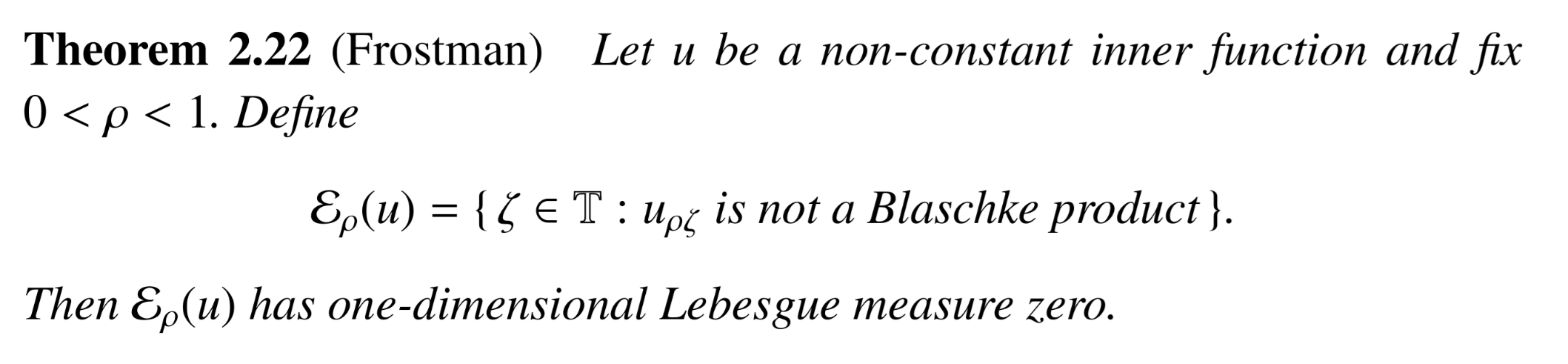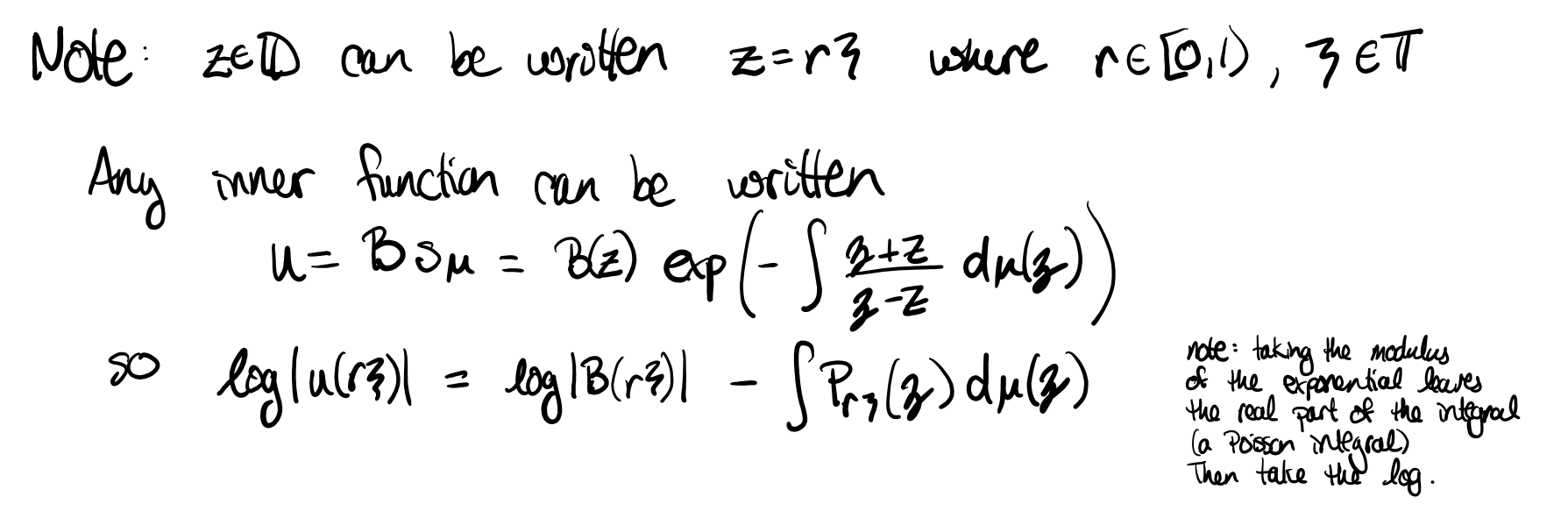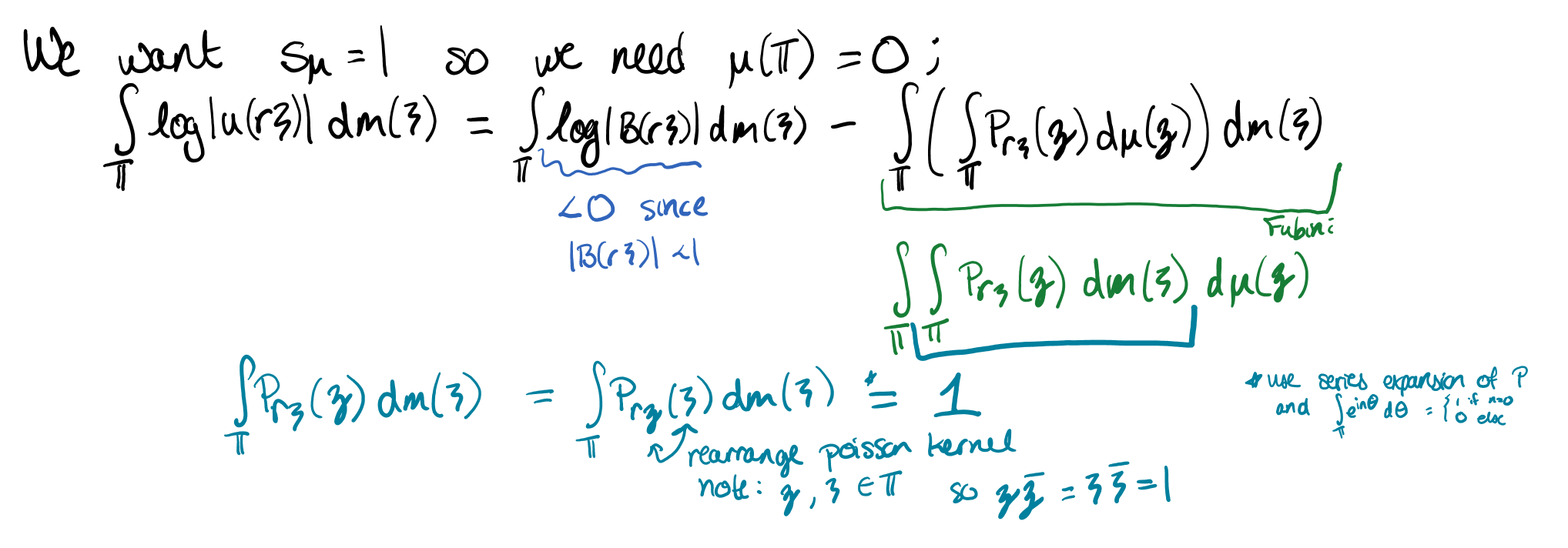### Frostman's Theorem surprises me

In my last post, I went into some detail about inner functions, Blaschke products, and singular inner functions.

The interesting thing about inner functions that I'll care about here is how they act when you compose them with an automorphism of the disk. This is called a Frostman shift:Frostman shifts continue to be inner functions: inner functions have boundary value modulus 1 and Mobius transforms take the circle to the circle, so the composition also has boundary values modulus 1.

What's WILD to me is that for "most" mobius transforms, the Frostman shift of ANY inner function will be a Blaschke product! This surprises me because Blaschke products have zeros in the disk and singular inner functions are zero free in the disk. The proof that Garcia, Mashreghi, and Ross provide is measure theoretic and broadly speaking, it works by showing that the measure for the singular inner part of a Frostman shift is zero (so when integrated and exponentiated, the singular inner factor is just 1).I think it's helpful to kind of think through the proof backwards here, since the way it's presented, some integral estimates done at the beginning seem irrelevant. We want to show that the singular inner factor is 1, so we need to show that the integral is zero. This means we need to do some logarithms to get the integral out of the exponential.Through some Fubini trickery and noting that (since $$B$$ is an inner function and therefore bounded by 1) $$\log|B(r\zeta)|<0$$, we get that the goal is to show